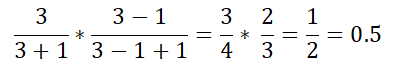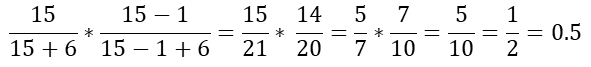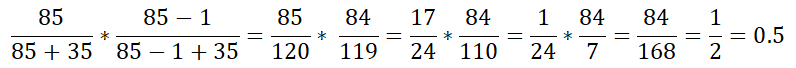# Exact division but can't satisfy the condition

## Recommended Posts

Hello,

I'm checking a formula as the following: (x / (x+y)) / ((x-1) / (x-1+y))With this calculation, I'm trying to get exactly 0.5 (not 4.9999, not 5.0000001). So, I have my code as:

```;Local \$x = 3, \$y = 1
;Local \$x = 15, \$y = 6
Local \$x = 85, \$y = 35

\$result = (\$x / (\$x + \$y)) * ((\$x - 1) / (\$x - 1 + \$y))
ConsoleWrite("Output will be: " & \$x & " " & \$y & " --> " & \$result & @CRLF)
If \$result = 0.5 Then
ConsoleWrite("YES, we're inside IF" & @CRLF)
Else
ConsoleWrite("Noooo" & @CRLF)
EndIf```

If I use the combination of (3,1) and (15,6) the result is exactly 0.5 and I can see the console "YES, we're inside IF". But the combination of (85,35) returns "Noooo".

We can also see that all these three combinations are exactly 0.5:If I change the condition as below, then yeah, it will return. But I'm looking for the exact 0.5 value.

```;If \$result = 0.5 Then
If \$result > 0.4999999999 Then```

So, my question is: Why the combination of (85,35) didn't return "YES, we're inside IF" even the output shows as it's exactly 0.5?

Notes / assumptions:

• We only support up to 15 decimals. These rational numbers are cut at 15th digit, so we can never calculate the exact value. This assumption can be wrong. Because:

(3,1)'s 2nd multiplier (2/3) will return 0.666666666666667; but we're inside that if.

(15,6)'s 2nd multiplier (14/20) will return 0.714285714285714; but we're inside that if again.

(85,35)'s 1st multiplier (85/120) will return 0.708333333333333 and 2nd multiplier (84/119) will return 0.705882352941177; but we're not inside that if anymore.

• If I add a rounding with 15 digits, it will work:
`\$result = Round((\$x / (\$x + \$y)) * ((\$x - 1) / (\$x - 1 + \$y)), 15)`

TY.

##### Share on other sites

5 minutes ago, taylansan said:

Why the combination of (85,35) didn't return "YES, we're inside IF" even the output shows as it's exactly 0.5?

Because it is not exactly 0.5.
It is only rounded on output, so you don't see this.
Consolewrite basically makes an implicit string() around the number variable to be output.
And this has the effect of rounding if the difference occurs after the 14th decimal place.
Modify your output line as follows to avoid this implicit rounding:

`ConsoleWrite(StringFormat("Output will be: %d %d --> %.17f\n", \$x, \$y, \$result))`

##### Share on other sites

No computer can calculate exactly 2/3 (as your first example).  A true mathematician would create a computer formula that fits the limitations of a machine.

“They did not know it was impossible, so they did it” ― Mark Twain

Spoiler

##### Share on other sites

Just a thought : when using OP's formula, we got 2 divisions first, then 1 final multiplication, which increases the resulting decimal issue :

`\$result = (\$x / (\$x + \$y)) * ((\$x - 1) / (\$x - 1 + \$y))`

Why not multiplying twice in the first place, then dividing only once ?

`\$result = (\$x * (\$x - 1)) / ((\$x + \$y) * (\$x - 1 + \$y))`

##### Share on other sites

Pixelsearch's answer is interesting and might be good enough. If you do need to verify that a number is exactly 1/2 though, you could do something like this...

```; Top half of the division
\$numerator = (\$x * (\$x - 1))
; Bottom half of the division
\$denomenator = ((\$x + \$y) * (\$x - 1 + \$y))

; Get the factors of the numerator and denomenator
Local \$aNumFactors = Factor(\$numerator) ; Factor isn't a function, left to you to figure out
Local \$aDenFactors = Factor(\$denomenator) ; Returns an array of numbers

; Now, compare the arrays and remove non-unique elements (I'm not doing this, it'd be non-trivial to write. Check on the _Array* functions?)

; Then, check to see if you have nothing in \$aNumFacors (it's a 1)
; and if you have a 2 remaining in \$aDenFacors```

All my code provided is Public Domain... but it may not work.Use it, change it, break it, whatever you want.

Spoiler

My Humble Contributions:
Personal Function Documentation - A personal HelpFile for your functions
Acro.au3 UDF - Automating Acrobat Pro
ToDo Finder - Find #ToDo: lines in your scripts
UI-SimpleWrappers UDF - Use UI Automation more Simply-er
KeePass UDF - Automate KeePass, a password manager
InputBoxes - Simple Input boxes for various variable types

##### Share on other sites

On 1/19/2023 at 8:59 PM, taylansan said:

(x / (x+y)) / ((x-1) / (x-1+y))

This is not what you use subsequently. You use (x/(x + y))*((x - 1)/(x + y - 1))

As @pixelsearchadvised, compute separately numerator and denominator, and only then perform the final division. Note that this is essentially what you show in your examples in the picture joined.

Since you only expect a result exactly = 1/2, you should make your life easier by computing the inverse fraction ((x + y)/x)*((x + y - 1)/(x - 1)) in the form ((x + y)*(x + y - 1))/(x*(x - 1)) and comparing to 2. Your computation remains then in ℤ unless the result != 2

```For \$x = 1 To 1000
For \$y = 1 To 1000
If ((\$x + \$y)*(\$x + \$y - 1))/(\$x*(\$x - 1)) = 2 Then
ConsoleWrite("(" & \$x & ", " & \$y & ") verifies the condition" & @LF)
EndIf
Next
Next```
```(3, 1) verifies the condition
(15, 6) verifies the condition
(85, 35) verifies the condition
(493, 204) verifies the condition```

Note also you can spare the division:

```For \$x = 1 To 1000
For \$y = 1 To 1000
If ((\$x + \$y)*(\$x + \$y - 1)) = 2*(\$x*(\$x - 1)) Then
ConsoleWrite("(" & \$x & ", " & \$y & ") verifies the condition" & @LF)
EndIf
Next
Next```

Edited by jchd

This wonderful site allows debugging and testing regular expressions (many flavors available). An absolute must have in your bookmarks.
RegExp tutorial: enough to get started
PCRE v8.33 regexp documentation latest available release and currently implemented in AutoIt beta.

SQLitespeed is another feature-rich premier SQLite manager (includes import/export). Well worth a try.
SQLite Expert (freeware Personal Edition or payware Pro version) is a very useful SQLite database manager.
An excellent eBook covering almost every aspect of SQLite3: a must-read for anyone doing serious work.
SQL tutorial (covers "generic" SQL, but most of it applies to SQLite as well)
A work-in-progress SQLite3 tutorial. Don't miss other LxyzTHW pages!
SQLite official website with full documentation (may be newer than the SQLite library that comes standard with AutoIt)

## Create an account

Register a new account

• ### Recently Browsing   0 members

×

• Wiki

• Back

• #### Beta

• Git
• FAQ
×
• Create New...